Name:    Conversion and Measurement Practice test/study guide

Matching

Match each item with the correct statement below.
 a. mass c. Celsius temperature scale b. Kelvin temperature scale d. weight

1.

kilogram is the SI base unit for this quantity

2.

the non-SI scale for temperature

3.

the SI scale for temperature

4.

the force of gravity on an object

Multiple Choice
Identify the choice that best completes the statement or answers the question.

5.

Multiply 5.0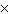10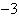and 8.010and report the answer in CORRECT scientific notation.
 a. 40100 b. 4.010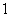c. 4010d. 4.0106.

The density of mercury is 5,427 kg/(m3). If the density of water is 1.0 g/mL, will mercury float or sink in water?
 a. Mercury will sink because the density of mercury is 5.427 g/mL, which is greater than the 1.0 g/mL density of water. b. Mercury will float because the density of mercury is 0.005427 g/mL, which is less than the 1.0 g/mL density of water. c. Mercury will sink because the density of mercury is 5,427 g/mL, which is greater than the 1.0 g/mL density of water. d. Mercury will float because the density of mercury is 0.05427 g/mL, which is less than the 1.0 g/mL density of water.

7.

What is the volume of 80.0 g of ether if the density of ether is 0.70 g/mL?
 a. 8.0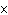10b. 1.110c. 8.810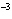d. 5.6108.

Which of the following conversion factors would you use to change 18 kilometers to meters?
 a. 1 km/1000 m c. 1000 m/1 km b. 100 m/1 km d. 1 km/100 m

9.

The standard base unit for mass is the
 a. gram. b. kilogram. c. meter. d. cubic centimeter.

10.

On the Celsius scale, at what temperature does water boil?
 a. 0° c. 100° b. 212° d. 32°

11.

Which of the following mass units is the largest?
 a. 1 ng b. 1 cg c. 1 mg d. 1 dg

12.

A conversion factor that shows the relationship between grams and kilograms is
 a. ÷ 1000 b.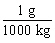c.1000 d.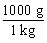13.

Which is the correct measurement for gray bar shown below?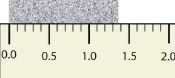a. 1.365 b. 1.36 c. 1.036 d. 1.3

14.

A change in the force of gravity on an object will affect its
 a. weight. b. kinetic energy. c. mass. d. density.

15.

In the lab, a student measured the length of an item to be 1.53 m, which digit is the estimated digit?
 a. none are estimated c. 1 b. 3 d. 5

16.

What is 1103 / 410-3 in CORRECT scientific notation? [divide]
 a. .25100 b. 2.5106 c. 2.510-5 d. 2.5105

17.

An object has a mass of 26.94 grams and a volume of 2.568 cubic centimeters. What material is it likely to be made of?
 Substance iron gold silver copper Density (g/cm3) 7.874 19.32 10.49 8.92
 a. Silver b. Gold c. Iron d. Copper

18.

The symbol mm represents a
 a. micrometer. c. manometer. b. megameter. d. millimeter.

19.

The symbols for units of length in order from largest to smallest are
 a. m, cm, mm, km. c. km, mm, cm, m. b. km, m, cm, mm. d. mm, m, cm, km.

20.

The SI base unit used to measure mass is the
 a. kilogram. c. meter. b. second. d. liter.

21.

How is 0.00099 written in scientific notation?
 a. 99 ´ 10–5 b. 9.9 ´ 104 c. 0.99 ´ 10–3 d. 9.9 ´ 10–4

22.

Which pair of quantities determines the density of a material?
 a. volume and weight c. volume and mass b. volume and concentration d. mass and weight

23.

Which safety measure should you take for every single laboratory experiment?
 a. Follow your teacher’s instructions. b. Open all classroom windows. c. Wear a lab apron. d. Use heat-resistant gloves.

24.

Express the product of 4.010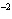m and 8.110m using the correct number of significant digits.
 a. 310b. 3.010c. 3.2410d. 3.21025.

All of the following are examples of units except
 a. kilometer. b. mass. c. ounce. d. gram.

26.

A beaker contains 0.5 L of water. What is the volume of this water in milliliters (1 L = 1000 mL)?
 a. 0.5 mL b. 5 mL c. 5000 mL d. 500 mL

27.

Which of the following shows good precision but poor accuracy?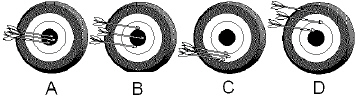a. C b. A c. D d. B

28.

The product of (2 ´ 104) x (4 ´ 10–6), expressed in scientific notation is ____.
 a. 6 ´ 10-24 b. 8 ´ 1010 c. 8 ´ 10–2 d. 8 ´ 10–24

29.

The density of aluminum is 2.70 g/cm3. What is the mass of a solid piece of aluminum with a volume of 1.50 cm3?
 a. 0.556 g b. 4.05 g c. 4.20 g d. 1.80 g

30.

Which of these is not an SI base unit?
 a. second b. kilogram c. liter d. Kelvin

31.

The SI base units for length and time are
 a. centimeter and hour. c. meter and second. b. centimeter and second. d. meter and hour.

32.

What is the density of an object having a mass of 8.0 g and a volume of 25 cm?
 a. 0.32 g/cmb. 2.0 g/cmc. 200 g/cmd. 3.1 g/cm33.

100  is equal to
 a. 10 b. 100 c. 1 d. 0

34.

The chief advantage of the metric system over other systems of measurement is that it ____.
 a. is in multiples of 10 b. is in multiples of 15 c. is derived from nature itself d. has more units

35.

The diameter of a carbon atom is 0.000 000 000 154 m. What is this number expressed in scientific notation?
 a. 1.5410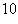m b. 1.5410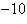m c. 1.5410m d. 1.5410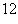m

36.

The unit m3 measures
 a. length. b. volume. c. area. d. time.

37.

The radius of Earth is 6 370 000 m. Express this measurement in km in scientific notation with the correct number of significant digits.
 a.km c.km b.km d.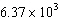km

38.

Which is the correct measurement for location marked by the arrow?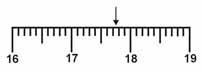a. 17.8 b. 17.7 c. 17.76 d. 17.076

39.

The expression of 8336 in scientific notation is ____.
 a. 83.3610b. 8.33610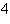c. 8.33610d. 8.3361040.

The density of an object is calculated by
 a. dividing its volume by its mass. c. multiplying its mass times its volume. b. adding its mass to its volume. d. dividing its mass by its volume.